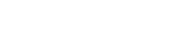# math.tanh

`FLOATmath.tanhFLOATx`

Available inall subroutines.

Computes the hyperbolic tangent of its argument `x`.

## Parameters

`x` - Floating point value representing a hyperbolic angle.

## Return Value

Upon successful completion, this function returns the hyperbolic tangent of `x`.

If `x` is `math.NAN`, a NaN will be returned.

If `x` is ±0, `x` will be returned.

If `x` is `math.POS_INFINITY` or `math.NEG_INFINITY`, ±1 will be returned.

If `x` is subnormal, a range error occurs and `x` will be returned.

## Errors

If the `x` argument is subnormal, then fastly.error will be set to `ERANGE`.

## Example

``````declare local var.fo FLOAT;
set var.fo = math.tanh(-1);``````

BETA